Next: Coherent noise filtering and Up: Proposed solutions to attenuate Previous: A filtering method

## A modeling approach

Instead of removing the noise by filtering (i.e., equation ()), the forward operatorcan be improved to model both noise and signal components. This technique treats the coherent noise as components of the data. Therefore, a coherent noise modeling operator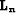and signal modeling operator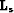are introduced to give Nemeth (1996)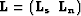in equation (). The model spacethen becomes the vector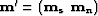, where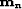is the model space for the noise andis the model space for the signal. Introducing an adequate noise modeling operator, the data residual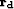will become IID and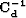can be approximated more safely with a diagonal operator with constant variance. In terms of fitting goal and omitting the regularization term, we have now, assuming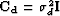whereis the identity matrix: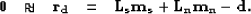(21)
The cost function becomes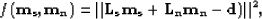(22)
and the estimated inverse for(see Appendixfor details)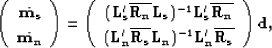(23)
withand. Both rows in equations () are the solutions of a weighted least-squares problem Menke (1989) for the following fitting goals:(24)
where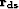and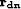are the residuals for the noise and signal components. Equation () is true becauseand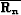are (1) projection operators, and (2) signal and noise filtering operators, respectively (see Appendixfor details). It is important to realize that in practice, the projection operators in equation () are not directly estimated andandare rather computed iteratively from the fitting goal in equation ().

What is interesting in equation (), however, is that the first fitting goal is very similar to equation (), the difference stemming from the choice of weighting (or filtering) operator. The modeling approach can be then interpreted as a weighting of the data residual with a projection filter that annihilates coherent noise. Therefore, the noise covariance matrix is approximated as follows: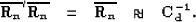(25)
To summarize, the filtering approach attempts approximating the noise covariance operator with prediction-error filters, thus using the property that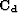is the power spectrum of the noise. In contrary, the modeling approach tackles the noise problem at its source by trying to model both the noise and signal simultaneously. Nonetheless, this technique can still be seen as a way of approximatingwith projection filters (i.e., equation ()).

Numerous authors Abma and Claerbout (1995); Ozdemir et al. (1999); Soubaras (1994); Soubaras (1995); Abma (1995) have proved that projection filters were more desirable for signal/noise separation than simple prediction-error filters. The main reason is that the spectrum of projection filters is in the range of zero to one. Therefore, the modeling approach should be used as much as possible for coherent seismic noise attenuation. As an illustration, Chapterdemonstrates on an interpolation problem of noisy data the benefits of the modeling approach compared to the filtering one.

In the next section, practical considerations are addressed for both noise filtering and noise modeling approaches. In particular, strategies for choosing the filters and operators are detailed. In addition, a pattern-based approach for signal/noise separation is briefly introduced.Next: Coherent noise filtering and Up: Proposed solutions to attenuate Previous: A filtering method
Stanford Exploration Project
5/5/2005